# Decomposition of Primes

Fix a prime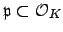and write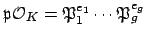, so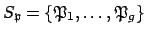.

Definition 13.2.1 (Residue class degree)   Suppose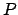is a prime of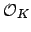lying over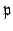. Then the ofis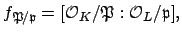i.e., the degree of the extension of residue class fields.

If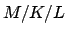is a tower of field extensions and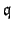is a prime of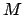over, then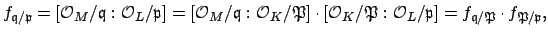so the residue class degree is multiplicative in towers.

Note that if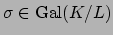and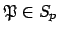, then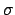induces an isomorphism of finite fields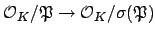that fixes the common subfield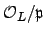. Thus the residue class degrees ofand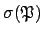are the same. In fact, much more is true.

Theorem 13.2.2   Suppose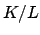is a Galois extension of number fields, and letbe a prime of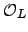. Write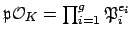, and let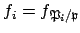. Then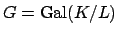acts transitively on the set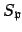of primes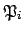,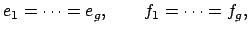and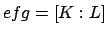, where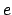is the common value of the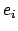and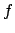is the common value of the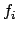.

Proof. For simplicity, we will give the proof only in the case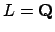, but the proof works in general. Suppose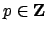and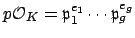, and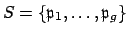. We will first prove that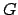acts transitively on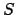. Let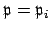for some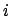. Recall that we proved long ago, using the Chinese Remainder Theorem (Theorem 9.1.3) that there exists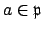such that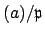is an integral ideal that is coprime to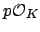. The product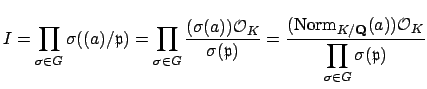(13.1)

is a nonzero integralideal since it is a product of nonzero integralideals. Sincewe have that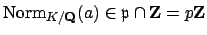. Thus the numerator of the rightmost expression in (13.2.1) is divisible by. Also, becauseis coprime to, each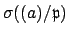is coprime toas well. Thus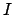is coprime to. Thus the denominator of the rightmost expression in (13.2.1) must also be divisibly byin order to cancel thein the numerator. Thus for anywe have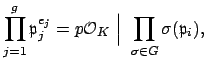which in particular implies thatacts transitively on the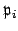.

Choose some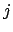and suppose that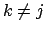is another index. Becauseacts transitively, there exists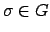such that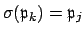. Applyingto the factorization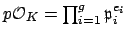, we see that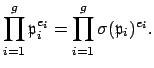Taking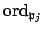on both sides we get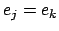. Thus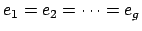.

As was mentioned right before the statement of the theorem, for anywe have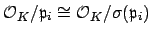, so by transitivity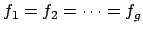. Sinceis a lattice in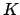, we have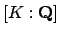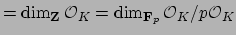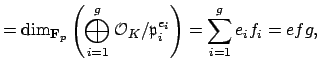which completes the proof.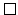The rest of this section illustrates the theorem for quadratic fields and a cubic field and its Galois closure.

Subsections
William Stein 2004-05-06